## Introduction

Soon after the discovery of photonic bandgap materials, it became known that electromagnetic modes could be localized on the surface of a photonic crystal (PhC).1,2 Such a state may exist if it cannot couple to any bulk state in the PhC or to any free-space mode in air; the bandgap of the PhC prohibits propagation, serving the same role as metals and negative-index materials in surface plasmon modes.3 These PhC surface states have been observed experimentally,4,5 and their localized properties have been applied to enhance light collimation6,7 and to manipulate photons on defects of the surface.5 It is often thought that bona fide photonic surface states can only exist below the light cone of the ambient air (i.e., below the continuum of radiation modes in air), where they are confined by total internal reflection. We show that, under appropriate but general conditions, photonic surface states may also exist inside the radiation continuum. Although coupling to radiation modes is allowed, such a state can have an infinite lifetime because different leakage channels interfere destructively to completely cancel each other.

To explain the physical origin of perfect cancellation among different leakage channels, we carry out rigorous analysis using temporal coupled-mode theory, which provides a generic description for coupling between the localized and propagating modes. We find that in addition to periodicity along the surface and a photonic bandgap that perfectly reflects light, the existence of these embedded Bloch surface eigenstates also requires unbroken time-reversal symmetry and a C2 rotational symmetry about the surface normal. The analysis applies to general wave systems, suggesting that this phenomenon may exist beyond optical systems. Such states offer new possibilities that may find use in the design of narrow-band waveguiding structures or in applications where strongly localized fields are desired.

We also note that, a lossless Bloch surface mode inside the radiation continuum falls within a rare class of states known as “embedded eigenvalues”.8,9,10,11,12,13,14,15,16,17,18,19,20,21,22,23,24,25,26,27,28,29,30,31,32,33,34,35,36,37,38 Shortly after the emergence of quantum mechanics, von Neumann and Wigner proposed that the single-particle Schrödinger equation may possess spatially localized states lying above the asymptotic limit of the potential and embedded in the continuum of extended states.8 Unlike non-embedded eigenvalues, embedded eigenvalues are typically difficult to find and generally disappear (become resonances) when slightly perturbed. Moreover, the impractical nature of the original proposed artificial Hamiltonians has made experimental verification difficult. For this reason, an embedded eigenvalue in quantum systems has never been demonstrated. The only known attempt concerns an electron bound state lying above the quantum-well potentials of a superlattice heterostructure, but within the bandgap of its mini bands,39 i.e., not an embedded eigenvalue. Thus, theoretical explorations to discover simpler, easily realizable, and more robust systems that can contain embedded eigenvalues are of great interest. Embedded eigenvalues have also been explored in Maxwell’s equations17,18,19,20,21,22,23,24,25,26,27,28,29 and in the acoustic and water wave equations.30,31,32,33,34,35,36,37,38 One occasion is when the spectrum of the problem can be separated by space group symmetry and when an odd-symmetry bound state lies in the continuum spectrum of the even states.17,18,19,20,21,22,23,30,31,32,33,34 Embedded states that do not rely on symmetry separability have received much attention,10,11,12,13,14,15,16,24,25,26,27,28,29,35,36,37,38 but have never been experimentally verified, primarily because they are fragile to perturbations. In this work, we identify theoretically a new realization of an embedded eigenvalue in a PhC system that does not rely on symmetry, yet should be easily realizable. Moreover, we find that unlike most known embedded eigenvalues, the one described here is robust from changes of system parameters; the eigenvalue simply shifts to another wavevector without fading into a resonance (as is characteristic in other embedded eigenvalue systems). The possibility of an embedded eigenvalue in a very similar structure was previously suggested by numerical investigations,40 but without proof or explanation of how such a state might arise.

## Surface eigenstate in the continuum

For comparison, we begin with a known example of a PhC surface-mode band structure,1,4 given in Figure 1a, where the PhC is a two-dimensional square lattice of dielectric cylinders. By terminating the surface rods in half on the (100) surface, one creates surface modes in the lowest photonic bandgap of TM modes (where electric field is normal to the plane,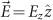). These states are on the lowest-frequency band of the surface rods; they lie below the light cone, and do not interact with the continuum of free-space modes. In comparison, higher-frequency bands of the surface rods can be brought into the photonic bandgap of the same bulk PhC by increasing the radii of the surface rods; this band structure is shown in Figure 1b. Here, the second band enters the light cone, where it couples to the radiation modes and becomes leaky. From finite-difference time-domain (FDTD) simulations,41 we excite these resonances with point sources on the surface, and perform harmonic analysis to obtain the lifetime τ and quality factor Q=ωτ/2 of these resonant modes. As shown in Figure 1c, there is a sharp increase of lifetime that approaches infinity when the surface-parallel wavevector is near ky≈0.28×2π/a, with a being the period of the PhC. In other words, there is a discrete point of wavevector where the resonant mode decouples from radiation and becomes an eigenstate. Figure 1d–1f show the field profiles Ez of the surface modes at and away from the peak. There is no leakage to air at the particular wavevector (Figure 1e), in contrast to nearby resonant modes (Figure 1d and 1f) where radiation leakage is clearly visible.

This phenomenon can be understood as an interference effect. The surface rods support a localized mode, which leaks to the right into the air (channel one), and to the left into the bulk PhC. Since the frequency of the state is inside the bandgap of the bulk PhC, all the left-going light is reflected, and part of that is transmitted through the surface rods into the air (channel two), interfering with channel one. When waves in these two channels have the same magnitude and differ in phase by π, destructive interference eliminates the radiation loss.

Periodicity is an important ingredient to these unique embedded states. If the structure is uniform in the surface-parallel direction (such as a plain uniform slab next to a uniform bulk structure), then any state within the radiation continuum must be leaky because fields in the air consist of propagating planewaves only. On the other hand, when the structure has periodicity in the surface-parallel direction (y-direction), fields in the air can consist of evanescent fields with any wavevector ky in the reciprocal lattice (i.e., ky’s that differ by integer multiples of 2π/a), so an infinite-lifetime resonance may exist. In the present case, periodicity also gives rise to band folding, which creates the resonant modes we study.

Given periodicity, it still remains to be answered if a perfect cancellation between the forward leakage and the back-reflected leakage can occur at all, and if so, what the other required conditions are. Furthermore, for experimental demonstrations, it is also critical to know whether the occurrence of such unique states is robust from small differences between the theoretical structure and the fabricated structure. In the next section, we carry out an analysis using temporal coupled-mode theory to answer these questions and to explain why such states do indeed exist and should be readily observable experimentally.

## Coupled-mode theory analysis

In our intuitive understanding of cancellation, the coupling between the surface mode and the radiating modes is an essential element. Therefore, we apply temporal coupled-mode theory,1,42 which provides a simple analytical description for resonant objects weakly coupled to incoming and outgoing ports. Temporal coupled-mode theory has been widely used in a variety of resonator systems ranging from optical waveguides and cavities, electronic circuits, to mechanical and acoustic resonators. It works well in the weak-coupling regime (when Q=ω0τ0/2>>π); in practice, it is nearly exact when Q>30, which is the case for all examples considered in this work. In our system, ω0 is the frequency of the localized mode, and τ0 is its lifetime when coupled to planewave modes in air (without considering the back-reflection). Inside the bandgap, the bulk PhC reflects all incoming waves. Thus, we treat the bulk PhC as a perfectly reflecting mirror, as illustrated in Figure 2. The amplitude A of the localized mode evolves as dA/dt=(−0−1/τ0)A in the absence of input powers. When incoming planewaves are included, the temporal coupled-mode theory equation can be written in Dirac notation as Refs. 1, 42 and 43: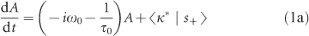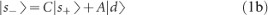where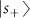and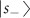are column vectors whose components s1+, s2+ and s1−, s2−, respectively, are amplitudes of the incoming and outgoing planewave modes as illustrated schematically in Figure 2, and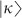and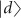are coupling coefficients between the localized mode and the planewave modes. The matrix C is a scattering matrix that describes the direct coupling between the planewave modes on both sides of the rods. We choose the normalizations such that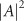is the energy inside the rods, and that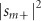and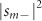are the incoming and outgoing powers in the mth planewave mode. The coupling coefficients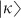,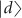and the direct scattering matrix C are not independent; energy conservation and time-reversal symmetry require these coefficients to be related by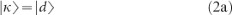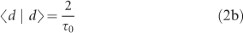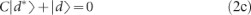as has been shown in Ref. 43.

The localized mode couples to one planewave mode on each side of the rods, with the surface-normal wavevector of the planewave given by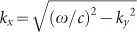. In the frequency range of interest, higher order diffractions (with ky differ by integer multiples of 2π/a) correspond to evanescent modes with imaginary kx that do not carry outgoing power, so they can be neglected. The reflection from the bulk PhC imposes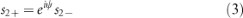with ψ being a phase shift. For reflection from the bulk PhC, there is no explicit expression of this phase shift. But for a closely related system where the PhC is replaced with a perfect metal distance l away from the rods, the phase shift is simply ψ=2kxlπ. Also, we write the direct scattering matrix as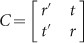where r [r′] and t [t′] are the complex reflection and transmission coefficients on the left [right] side of the rods. In the absence of input power (s1+=0), a stationary state with no leakage (s1−=0) occurs when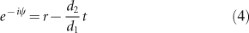This equation is a mathematical translation of the intuitive statement that the forward leakage d1A and the back-reflected leakage tes2− cancel each other.

The question remains whether such cancellation can occur generally, and if so, what are the requirements. Assume time-reversal symmetry in the system is unbroken (i.e., negligible absorption, and no magneto-optic effect imposed). Then, the complex conjugate of fields in the direct scattering process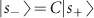is a solution of the Maxwell’s equations with opposite wavevector,1 namely,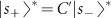where C′ is the scattering matrix of wavevector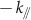. Further, assume that the structure has twofold rotational symmetry C2 about the surface normal, so that C=C′; in the present example where the z dimension is uniform, mirror symmetry σy is sufficient. These results combine to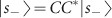, and so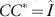, which gives the Stokes relations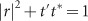and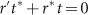. The same procedure on the process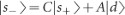leads to equation (2c), which reduces to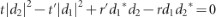. Combining this and the Stokes relations, we get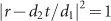. In other words, in the presence of time-reversal symmetry and a C2 rotational symmetry about the surface normal, the right-hand side of equation (4) always has unit magnitude.

The left-hand side of equation (4) also has unit magnitude because of the perfectly reflecting boundary. In such case, the magnitude condition is always satisfied, and equation (4) can be reduced to the phase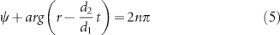with n being an integer. In other words, this phase condition, equation (5), is the only requirement for achieving perfect cancellation. Intuitively, it may be understood as a sum of phase shifts, with the first term ψ coming from propagation and reflection, and the second term arg (rd2t/d1) coming from coupling with the localized mode. A conceptually similar phase-shift equation has been used in the context of metallic nanorod cavity, where the second term is replaced with the phase shift from coupling with surface plasmon.44 Equation (5) comes down to locating where the phase shift crosses integer multiples of 2π. Such an intersection may be located by varying the wavevector ky; it results in discrete states with infinite lifetimes, as we have observed in Figure 1c. Not all bands in the light cone will produce such crossings; for example, our previous reasoning indicates that structures without periodicity cannot support such embedded states, so the phase shift for such structures should never cross integer multiples of 2π. But once a crossing is found, the existence of a root will be robust to changes of system parameters since the perturbation will only shift the intersection to a slightly different wavevector.

We note a subtle but important difference between the embedded eigenvalue we study here and that in most known examples. In most examples, the embedded eigenvalue disappears when the parameters of the system deviates slightly from the designed structure or potential, making it very difficult to observe experimentally. In our case, we are concerned with a family of eigenproblems, with each eigenproblem defined by the wavevector of the system. When the parameters of the system change, the embedded eigenvalue disappears from the eigenproblem of one wavevector, but appears in that of another wavevector. In experiments, states with all wavevectors can be measured, and therefore, this shifting does not prevent us from physically observing such states. Following this reasoning, we can also expect that embedded eigenvalues in other periodic systems such as Refs. 27 and 37 should also be robust to parameter changes.

Lastly, we mention that the lifetime of certain resonant cavities can be increased by optimizing their geometries to cancel the dominant component of the far-field radiation.45,46,47,48 However, the embedded eigenstate described here, though not localized in the surface-parallel direction, achieves complete cancellation, which includes all components of the far-field radiation.

## Validation of analysis

The coupled-mode analysis has translated our intuitive picture of cancellation into mathematical statements. Furthermore, it showed that perfect cancellation is possible given time-reversal and C2 symmetries in the system. Next, we compare its quantitative predictions with numerical simulations.

With no input power (s1+=0), equations (1) and (3) have solution A(t)=A(0)exp (−iωtt/τ) with decay rate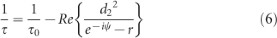This gives the lifetime τ and quality factor Q of the mode. At the perfect-cancellation points discussed in previous section, τ is infinite; away from these points, τ is finite.

Several reference quantities, the natural frequency ω0, lifetime τ0 and the left/right ratio of the decay rates which we denote as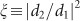, are measurable and can also be calculated in FDTD simulations. The direct scattering matrix C may be approximated by treating the surface rods as a slab. With these values known, the coupling coefficients d1 and d2 can be determined from equation (2), and lifetime of the localized mode and the location of the perfect-cancellation point can be evaluated from equations (6) and (5) respectively to yield quantitative predictions. Equation (2c) gives a quadratic equation with two roots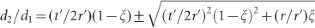; for rods with mirror symmetry in x direction, we have ξ=1 and r=r′, and these two roots correspond to modes whose Ez are even (d1= d2) or odd (d1=−d2) in x direction.

For quantitative validation of these predictions, we consider some explicit examples. First, we consider a simplifying example where the PhC is replaced with a perfect-metal boundary at distance l from the surface rods (Figure 3a inset). In this system, the phase shift at the reflection boundary is simply π, making evaluation of the coupled-mode theory equations easy. This perfect-metal boundary also makes the eigenmodes of this structure equivalent to the asymmetric eigenmodes of a double-column structure (with separation 2l), which has been studied in Ref. 27 with a Fabry–Pérot transmission analysis. Figure 3a shows the band structure for separation l=1.6a. The quality factor Q(ky) of the second band inside the light cone is computed from FDTD simulations and shown in circles in Figure 3b; two infinite-lifetime states corresponding to n=0 and n=1 in equation (5) are observed. Since ψ=2kxlπ, we expect that qualitatively the surface-normal wavevector kx of the infinite-Q states should be inversely proportional to the separation l, which is confirmed in Figure 3c. We also expect that at larger separation, there will be more embedded eigenstates that correspond to phase shifts differing by 2; this is indeed observed in the numerical simulations.